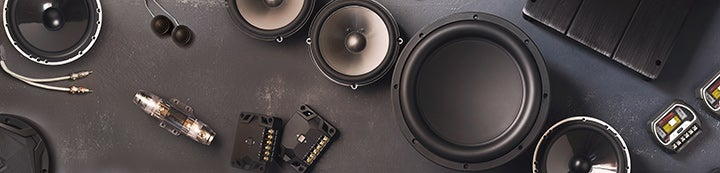1 - 4 of 4 Posts

#### blammo585

·
##### Registered
Joined
·
310 Posts
Discussion Starter
Here's something I've always wondered. Let's say you have two 2 ohm subs and a 2-channel amp that will put out 100 watts x 2 into 2 ohms or 200 watts x 1 into 4 ohms. If it's you, would you wire it up as 100 x 2, or are you going to wire the subs in series to make them a 4 ohm load and run it 200 x 1? I've always had this thought that 200 is more power, but if the two subs are sharing the power any way then would running them on their own channel be better? THD is always lower running in 2-channel mode than in bridged so in theory the 100 x 2 would be a little "cleaner", right?

Keep in mind, the power rating here is just an example. It could be anything, like 500 x 2 at 2 ohms and 1000 x 1 into 4.

#### dgage

·
##### Registered
Joined
·
1,994 Posts
Go with a single channel so that you know the amp is splitting the load and sending a single SHARED signal. It shouldn’t matter but bridging the channels will ensure that any differences between the two channels is shared so the two subs see identical signal and power. There is the possibility with two channels that there could be minor differences.

You didn’t ask it but I also like to build pairs of subs with a shared chamber so that the subs will be forced to work together in unison. Similar to two people walking together often get into step, you want the subs to play together and a shared airspace ensures their response is identical.

#### Fast Hot Rod

·
##### Registered
Joined
·
304 Posts
It's a good question, Blammo585. The part I think that you're missing is how the circuits actually behave, but maybe I can help explain it to you.

When you stop and think about the first setup, you have a 2-channel amp that can produce 100 watts x 2 at 2 ohms. That means that the total output is 100 watts x 2 = 200 watts total.

In the second setup, you have 200 watts being delivered into two subs... so each sub only gets 100 watts each. Here's how the math breaks down:

Using Ohms Law and looking at the first way of doing it:

Power = Voltage * Amperes
Voltage = Amperes * Ohms

Using substitution: Power = (Amperes * Ohms) * Amperes
Power = Amperes ^2 * Ohms
(Amperes ^2 is just Amperes Squared, or Amperes * Amperes.)

So if you have 100 watts into 2 ohms:

100 watts = Amperes ^2 * 2 Ohms
100/2 = Amperes ^2
50 = Amperes ^2
Square root of 50 = Amperes
7.07 = Amperes

So we plug that back into the equation to find the voltage:

Voltage = Amperes * Ohms
Voltage = 7.07 * 2
Voltage = 14.14

Check our math:

Watts = Voltage * Amperes
Watts = 14.14 * 7.07
Watts = 99.98 which is close enough. (Rounding errors.)

So when we look at the other way of doing it, we're bridging the two channels into a single channel. We're connecting the subs in series, so it's 2 Ohms + 2 Ohms for a 4 Ohm total load.

If the amp puts out 200 watts at 4 Ohms:

Watts = Amperes ^2 * Ohms
200 Watts = Amperes ^2 * 4 Ohms
200/4 = Amperes ^2
50 = Amperes ^2
Square root of 50 = Amperes
7.07 = Amperes

Notice that in both applications, the amplifier is putting out the same amount of Amperes. So what must be changing is the amplifier's Voltage. Let's check that one:

Voltage = Amperes * Ohms
Voltage = 7.07 * 4
Voltage = 28.28 which is twice the amount of it operating in single channel mode.

Checking our math:

Power = Voltage * Amperes
Power = 28.28 * 7.07
Power = 199.93 which is close enough. (Rounding errors.)

So if we wanted to, we could look at how the second setup is working... we know what the voltage is, we know what the current (Ampere) is through the wire, so we can calculate how much power is being used per sub.

On a series circuit, the current is the same. Each sub will 'see' 7.07 Amperes. For a fixed resistance of say 4 Ohms, and using Ohms Law:

Voltage = Amperes * Ohms
Voltage = 7.07 * 4
Voltage = 28.2...so that checks out. But if we look at each of those 2 Ohm subs separately:

Voltage = 7.07 * 2
Voltage = 14.14
Power = 14.14 * 7.07
Power = 99.96 which is close to 100 watts.

So each sub will be getting 100 watts no matter which way you decide to wire it.

Hope this helps.

Mark

·